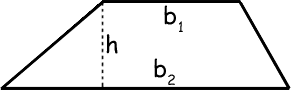MATHguide's Area of Trapezoids Quizmaster
Review the Lesson | MATHguide homepage Updated June 18th, 2023

 If b1 is the top base, b2 is the bottom base, and h is the height of the trapezoid,find the following information when b1 = 16 units, b2 = 21 units, and h = 4 units: What is the area?Atrapezoid = units2